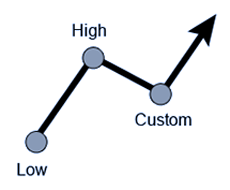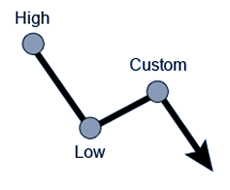# Fibonacci Calculator

The Fibonacci Calculator will calculate Fibonacci retracements and extensions based on three values (high, low, and custom value).

There are three main values that are used in the Fibonacci Online Calculator to figure out Fibonacci retracements and extensions. The foregoing values are high, low and custom.

There is a range of Fibonacci retracements that are available at the Fibonacci Online Calculator: 23.6%, 38.2%, 50%, 61.8%, 76.4%, 100%, and 138.2%.

In addition to the retracements, an array of the Fibonacci extensions are available to be used in the Fibonacci Calculator: 261.8%, 200%, 161.8%, 138.2%, 100%, and 61.8%.

The diagrams showing either the uptrend Fibonacci Retracements or downtrend Fibonacci Retracements can be displayed by the calculator. The points on the diagrams show low, custom or high values. The arrow at the end of the curve is showing the trend direction.

The results of the calculations are revealed under the diagrams with two columns, retracements and extensions.

Forex Fibonacci Calculator

Values

 Uptrend Fibonacci RetracementDowntrend Fibonacci RetracementResults
Retracements
0% (b)
23.6%
38.2%
50%
61.8%
76.4%
100% (a)
138.2%
Extensions
261.8%
200%
161.8%
138.2%
100%
61.8%